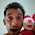### What Makefile am I in?

A common request when using GNU Make is: "Is there a way to find the name and path of the current Makefile?". By 'current' people usually mean that Makefile that GNU Make is currently parsing. There's no built-in way to quickly get the answer, but there is a way using the GNU Make variable MAKEFILE_LIST.

MAKEFILE_LIST (documented in the manual here) is the list of Makefiles currently loaded or included. Each time a Makefile is loaded or included the variable is appended. The paths and names in the variable are relative to the current working directory (where GNU Make was started or where it moved to with the -C or --directory option). The current working directory is stored in the CURDIR variable.

So you can quite easily define a GNU Make function (let's call it where-am-i) that will return the current Makefile (it uses \$(word) to get the last Makefile name from the list):
`where-am-i = \$(CURDIR)/\$(word \$(words \$(MAKEFILE_LIST)),\$(MAKEFILE_LIST))`

then whenever you want to find out the full path to the current Makefile write the following at the top of the Makefile (the 'at the top' part is important because any include statement in the Makefile will change the value of MAKEFILE_LIST so you want to grab the location of the current Makefile right at the top):
`THIS_MAKEFILE := \$(call where-am-i)`

Example:

Here's Makefile
`where-am-i = \$(CURDIR)/\$(word (\$words \$(MAKEFILE_LIST)),\$(MAKEFILE_LIST)include foo/Makefile`

foo/Makefile contains:
`THIS_MAKEFILE := \$(call where-am-i)\$(warning \$(THIS_MAKEFILE))include foo/bar/Makefile`

foo/bar/Makefile contains:
`THIS_MAKEFILE := \$(call where-am-i)\$(warning \$(THIS_MAKEFILE))`

Running this on my machine (with the first Makefile in /tmp) gives the output:

`foo/Makefile:2: /tmp/foo/Makefilefoo/bar/Makefile:2: /tmp/foo/bar/Makefile`Starting in GNU make 3.81, you can use \$(lastword \$(MAKEFILE_LIST)) instead of \$(word (\$words \$(MAKEFILE_LIST)),\$(MAKEFILE_LIST).Grokon said…

where-am-i = \$(CURDIR)/\$(word \$(words \$(MAKEFILE_LIST)),\$(MAKEFILE_LIST)

than

where-am-i = \$(CURDIR)/\$(word (\$words \$(MAKEFILE_LIST)),\$(MAKEFILE_LIST)

(parenthesis for words command is badly placed)Anonymous said…
it will not work after nested includes